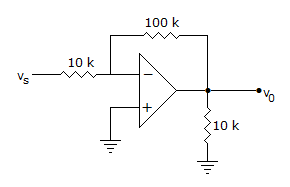# Electronics and Communication Engineering - Analog Electronics - Discussion

13.

The input impedance of op-amp circuit of figure is[A]. 120 k ohm [B]. 110 k ohm [C]. infinity [D]. 10 k ohm

Explanation:

Due to the presence of virtual ground at input, the resistance in the series path of input of inverting amplifier is input impedance.

 Chenelyn Cedron said: (Aug 3, 2016) Let's try some math and the Miller's Theorem to solve this one. First, the closed loop gain A{cl} of a negative feedback is: A{cl} = (V{out})/(V{in}) = (-Rf)/(Ri) Where Rf is the feedback resistance and Ri is the input resistance. It should be clear that Rf is equal to Z. Using Miller's theorem, Z{equivalent_input} = (Z)/(1 - A) = (Rf)/(1 - ((-Rf)/(Ri)). Z{equivalent_input} = ((Ri)(Rf))/(Ri + Rf). Z{equivalent_input} = ((10k)(100k))/(100k + 10k). Z{equivalent_input} = 9.09k. Letter D is the nearest choice.

 Jyothi R said: (Oct 4, 2016) By virtual ground concept. vs - (10k * is) = 0;. So, vs/is = input impedence = 10k ohm.

 Hindosh said: (Aug 2, 2017) Thank you both for the given explanation.

 Laksh said: (Mar 21, 2018) Wonderful explanation, thank you all.

 Pankhuri Saxena said: (Apr 4, 2018) Well said, thanks @ChenelynCedron.